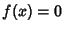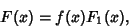## Abel's Irreducibility Theorem

If one Root of the equation, which is irreducible over a Field, is also a Root of the equationin, then all the Roots of the irreducible equationare Roots of. Equivalently,can be divided bywithout a Remainder,whereis also a Polynomial over.

Abel, N. H. Mémoire sur une classe particulière d'équations résolubles algébriquement.'' J. reine angew. Math. 4, 1829.